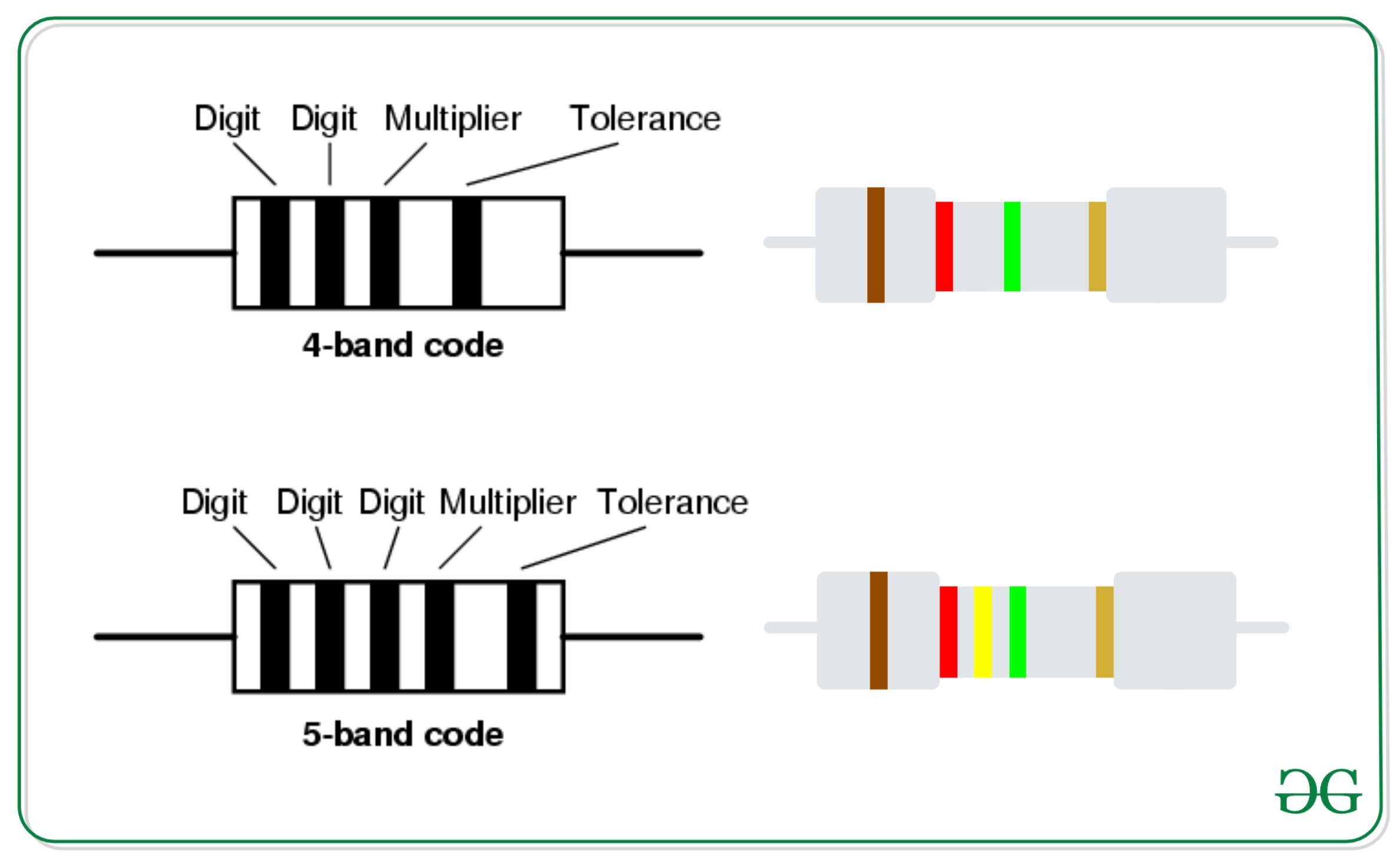# Program to calculate Resistance using given color code in circuits

There are many different types of Resistor available which can be used in both electrical and electronic circuits. The resistance value, tolerance, and wattage rating are generally printed onto the body of the resistor as color bands. The task is to find the resistance of 4-band resistor and 5-band resistor.### Program to find the resistance of 4-Band Resistor

Given four strings A, B, C and D which denotes the color codes of the 4-band resistor. The task is to find the resistance, tolerance and wattage rating using the given color codes.

Examples:

Input: A = “black”, B = “brown”, C = “red”, D = “green”
Output: Resistance = 01 x 100 ohms +/- 0.5 %

Input: A = “red”, B = “orange”, C = “yellow”, D = “green”
Output: Resistance = 23 x 10k ohms +/- 0.5 %

Approach: The idea is to store the digits and multiplers as in the hash-map and then the resistance of the resistor can be computed.

Below is the implementation of the above approach:

## Python3

 `# Python implementation to find the  ` `# resistance of the resistor with ` `# the given color codes ` ` `  `# Function to find the resistance  ` `# using color codes ` `def` `findResistance(a, b, c, d): ` `     `  `    ``# Hash-map to store the values  ` `    ``# of the color-digits ` `    ``color_digit ``=` `{``'black'``: ``'0'``, ` `                   ``'brown'``: ``'1'``,  ` `                   ``'red'``: ``'2'``, ` `                   ``'orange'``: ``'3'``,  ` `                   ``'yellow'``: ``'4'``, ` `                   ``'green'` `: ``'5'``,  ` `                   ``'blue'` `: ``'6'``, ` `                   ``'violet'` `: ``'7'``,  ` `                   ``'grey'` `: ``'8'``, ` `                   ``'white'``: ``'9'``} ` `     `  `    ``multiplier ``=` `{``'black'``: ``'1'``, ` `                  ``'brown'``: ``'10'``,  ` `                  ``'red'``: ``'100'``,  ` `                  ``'orange'``: ``'1k'``,  ` `                  ``'yellow'``: ``'10k'``,  ` `                  ``'green'` `: ``'100k'``,  ` `                  ``'blue'` `: ``'1M'``,  ` `                  ``'violet'` `: ``'10M'``,  ` `                  ``'grey'` `: ``'100M'``,  ` `                  ``'white'``: ``'1G'``} ` `     `  `    ``tolerance ``=` `{``'brown'``: ``'+/- 1 %'``,  ` `                  ``'red'` `: ``'+/- 2 %'``,  ` `                 ``'green'``: ``"+/- 0.5 %"``,  ` `                  ``'blue'``: ``'+/- 0.25 %'``,  ` `                 ``'violet'` `: ``'+/- 0.1 %'``,  ` `                  ``'gold'``: ``'+/- 5 %'``,  ` `                 ``'silver'` `: ``'+/- 10 %'``,  ` `                  ``'none'``: ``'+/-20 %'``} ` `     `  `    ``if` `a ``in` `color_digit ` `       ``and` `b ``in` `color_digit\ ` `       ``and` `c ``in` `multiplier  ` `       ``and` `d ``in` `tolerance: ` `           ``xx ``=` `color_digit.get(a) ` `           ``yy ``=` `color_digit.get(b) ` `           ``zz ``=` `multiplier.get(c) ` `           ``aa ``=` `tolerance.get(d) ` `           ``print``(``"Resistance = "``+``xx ``+` `yy``+``\ ` `                 ``" x "``+``zz``+``" ohms "``+``aa) ` `    ``else``: ` `        ``print``(``"Invalid Colors"``) ` `         `  `# Driver Code ` `if` `__name__ ``=``=` `"__main__"``: ` `    ``a ``=` `"black"` `    ``b ``=` `"brown"` `    ``c ``=` `"red"` `    ``d ``=` `"green"` `     `  `    ``# Function Call ` `    ``findResistance(a, b, c, d) `

Output:

```Resistance = 01 x 100 ohms +/- 0.5 %
```

### Program to find the resistance of 5-Band Resistor

Given five strings A, B, C, D and E which denotes the color codes of the 5-band resistor. The task is to find the resistance, tolerance and wattage rating using the given color codes.

Examples:

Input: A = “black”, B = “brown”, C = “red”, D = “green”, E = “silver”
Output: Resistance = 012 x 100k ohms +/- 10 %

Input: A = “red”, B = “orange”, C = “yellow”, D = “green”, E = “gold”
Output: Resistance = 234 x 100k ohms +/- 5 %

Approach: The idea is to store the digits and multiplers as in the hash-map and then the resistance of the resistor can be computed.

Below is the implementation of the above approach:

## Python3

 `# Python implementation to find the  ` `# resistance of the resistor with ` `# the given color codes ` ` `  `# Function to find the resistance  ` `# using color codes ` `def` `findResistance(a, b, c, d, e): ` `     `  `    ``# Hash-map to store the values  ` `    ``# of the color-digits ` `    ``color_digit ``=` `{``'black'``: ``'0'``, ` `                   ``'brown'``: ``'1'``,  ` `                   ``'red'``: ``'2'``, ` `                   ``'orange'``: ``'3'``,  ` `                   ``'yellow'``: ``'4'``, ` `                   ``'green'` `: ``'5'``,  ` `                   ``'blue'` `: ``'6'``, ` `                   ``'violet'` `: ``'7'``,  ` `                   ``'grey'` `: ``'8'``, ` `                   ``'white'``: ``'9'``} ` `     `  `    ``multiplier ``=` `{``'black'``: ``'1'``, ` `                  ``'brown'``: ``'10'``,  ` `                  ``'red'``: ``'100'``,  ` `                  ``'orange'``: ``'1k'``,  ` `                  ``'yellow'``: ``'10k'``,  ` `                  ``'green'` `: ``'100k'``,  ` `                  ``'blue'` `: ``'1M'``,  ` `                  ``'violet'` `: ``'10M'``,  ` `                  ``'grey'` `: ``'100M'``,  ` `                  ``'white'``: ``'1G'``} ` `     `  `    ``tolerance ``=` `{``'brown'``: ``'+/- 1 %'``,  ` `                  ``'red'` `: ``'+/- 2 %'``,  ` `                 ``'green'``: ``"+/- 0.5 %"``,  ` `                  ``'blue'``: ``'+/- 0.25 %'``,  ` `                 ``'violet'` `: ``'+/- 0.1 %'``,  ` `                  ``'gold'``: ``'+/- 5 %'``,  ` `                 ``'silver'` `: ``'+/- 10 %'``,  ` `                  ``'none'``: ``'+/-20 %'``} ` `     `  `    ``if` `a ``in` `color_digit ` `       ``and` `b ``in` `color_digit\ ` `       ``and` `c ``in` `color_digit ` `       ``and` `d ``in` `multiplier\ ` `       ``and` `e ``in`  `tolerance: ` `           ``xx ``=` `color_digit.get(a) ` `           ``yy ``=` `color_digit.get(b) ` `           ``zz ``=` `color_digit.get(c) ` `           ``aa ``=` `multiplier.get(d) ` `           ``bb ``=` `tolerance.get(e) ` `           ``print``(``"Resistance = "``+``xx ``+` `yy\ ` `               ``+` `zz``+``" x "``+``aa``+``" ohms "``+``bb) ` `    ``else``: ` `        ``print``(``"Invalid Colors"``) ` `         `  `# Driver Code ` `if` `__name__ ``=``=` `"__main__"``: ` `    ``a ``=` `"red"` `    ``b ``=` `"orange"` `    ``c ``=` `"yellow"` `    ``d ``=` `"green"` `    ``e ``=` `"gold"` `     `  `    ``# Function Call ` `    ``findResistance(a, b, c, d, e) `

Output:

```Resistance = 234 x 100k ohms +/- 5 %
```

Attention reader! Don’t stop learning now. Get hold of all the important DSA concepts with the DSA Self Paced Course at a student-friendly price and become industry ready.

My Personal Notes arrow_drop_upCheck out this Author's contributed articles.

If you like GeeksforGeeks and would like to contribute, you can also write an article using contribute.geeksforgeeks.org or mail your article to contribute@geeksforgeeks.org. See your article appearing on the GeeksforGeeks main page and help other Geeks.

Please Improve this article if you find anything incorrect by clicking on the "Improve Article" button below.